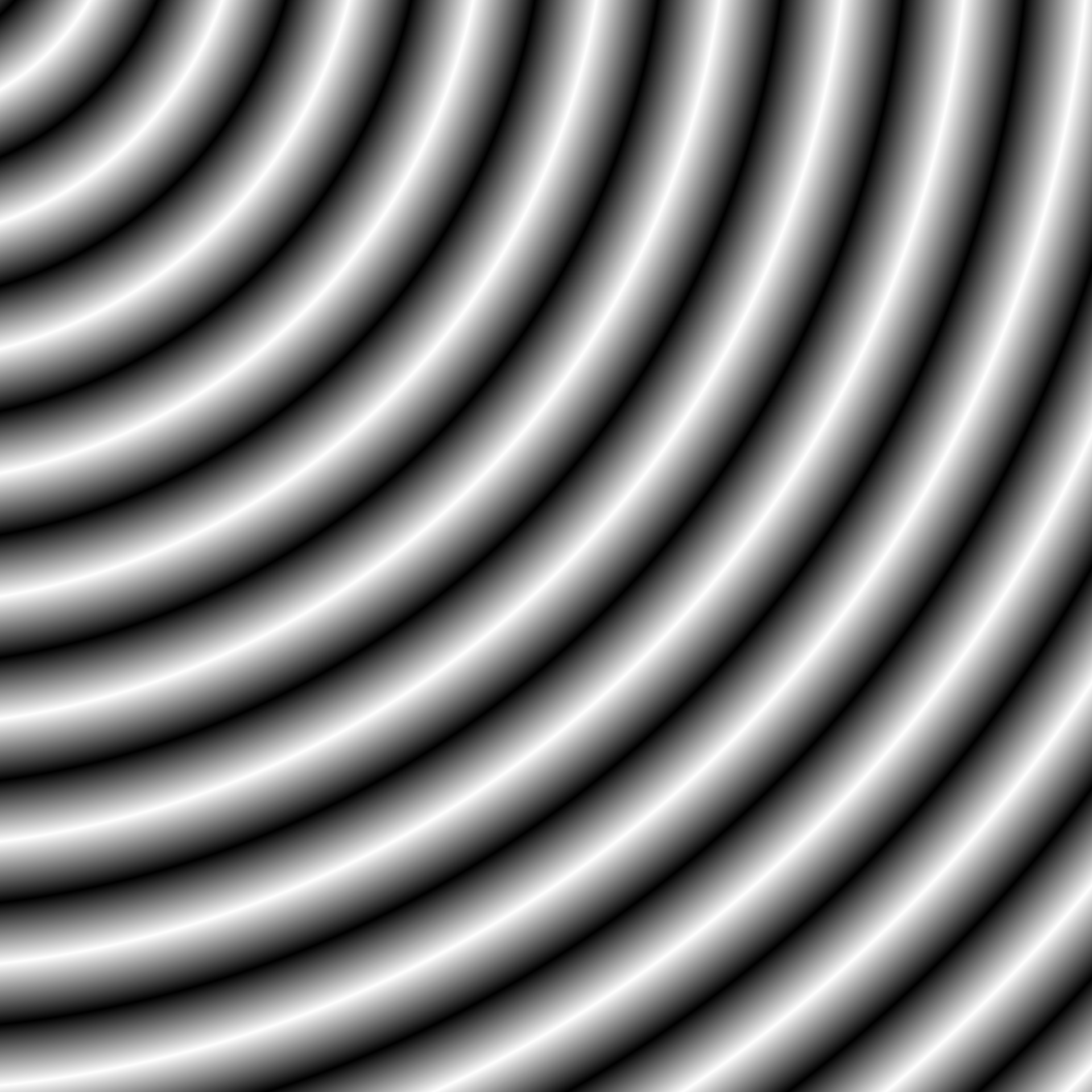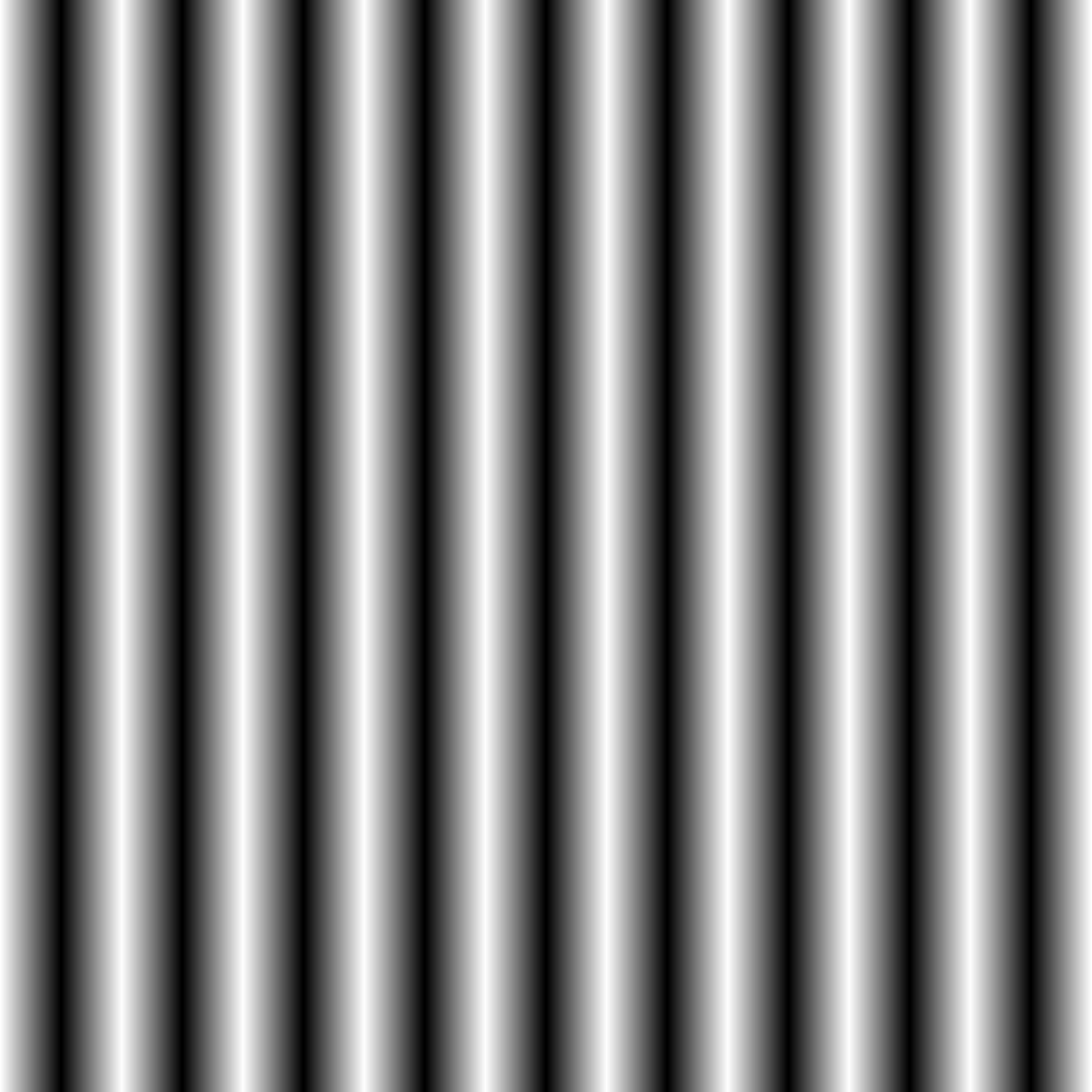This generator creates a pattern of concentric circles centered at 0. Depending on how many dimensions you supply it can be used to generate cylinders and circles as well. The output value is the shortest distance to the nearest sphere normalised to be between -1 and 1. The frequency determines the radius multiplier for each unit sphere.

gen_spheres(x, y = NULL, z = NULL, t = NULL, frequency = 1, ...)

## Arguments

x, y, z, t The coordinates to get pattern from The frequency of the generator ignored

## Value

A numeric vector

Other Pattern generators: gen_checkerboard(), gen_waves()

## Examples

grid <- long_grid(seq(1, 10, length.out = 1000), seq(1, 10, length.out = 1000))
grid$circles <- gen_spheres(grid$x, grid$y) grid$cylinders <- gen_spheres(grid\$x)

plot(grid, circles)plot(grid, cylinders)